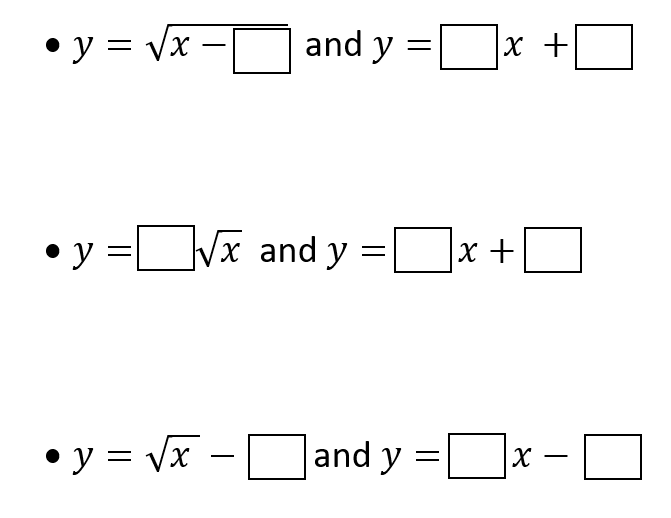# Radical And Linear Function Intersection

Directions: Using the digits 1 to 9 at most one time each, to make one set of functions intersect exactly twice, one set of functions intersect exactly once, and one set of functions never intersect.### Hint

How do transformations affect each function? If solving algebraically, what happens to get 1 solution? 2 solutions? No solution?